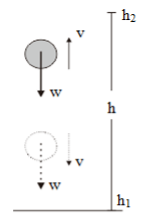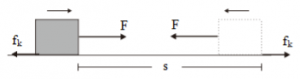# Conservative force and nonconservative force

1. Conservative Force

1.1 Weight (w)Observe an object which moves vertically upwards until reaching a maximum height before moving downwards towards its initial position. When moving vertically upwards by h, the weight is opposite in direction from displacement. Thus, the weight does negative work on the object.

W = w h (cos 180o) = – w h = – m g h

After reaching a maximum height, the object moves downwards towards its initial position by h. When moving downwards, the weight is in the same direction as the displacement. Because it is in the same direction as displacement, the weight does positive work.

W = w h (cos 0o) = w h = m g h

The object’s mass (m), gravitational acceleration (g), and height (h) are the same, so the work done by the weight since the object starts moving vertically upwards until it returns to its initial position is zero.

W = m g h – m g h = 0

[irp]

1.2 Spring Force

Observe a spring placed in a horizontal position. If the right end of the spring is pushed or compressed to the left, the spring exerts a thrust force to the right. You can prove this by compressing a spring. For instance, place an object on the right end, then press the object to the left. After the spring deviates by Δx, remove your hand from the object and the spring. When the hand is no longer in contact with the spring, the spring will push the object back to the right.

When the object moves to the left, the direction of its motion and displacement are opposite to the direction of the spring’s force. Because the directions are opposite to each other, the spring’s force does negative work.

W = – ½ k (Δx)2

When the object moves to the right, the direction of its motion and displacement is the same as the spring’s force. Because the directions are the same, the spring’s force does positive work.

W = ½ k (Δx)2

The spring used is the same, so the spring’s constant (k) is the same. The spring deviation (Δx) is also the same.

Thus, the work done by weight when the object starts to move to the left by Δx and then moves back to the right by Δx is zero.

W = ½ k (Δx)2 – ½ k (Δx)2 = 0

The work done by weight and spring force since the object starts to move from its initial position until it returns there is equal to zero. If the work was done by a force since it moves from its initial position until it returns there is equal to zero, the force is called conservative force. As such, spring force and weight are examples of conservative forces.

[irp]

2. Non-Conservative Force

Observe an object which is pushed to the right and then pushed back to the left. When the object is moving or displaced to the right, the direction of the object’s displacement is the same as the direction of thrust force (F) and opposite to the direction of kinetic frictional force (fk). As it is in the same direction as displacement, the thrust force does positive work on the object.W = F s

On the contrary, the kinetic frictional force does negative work on the object.

W = – fk s

The work was done by the thrust force and frictional force on the object when the object starts to move from its initial position until it returns there is:

W = 2 F s

W = -2 fk s

The work done by the thrust force and kinetic frictional force since the object starts to move from its initial position until it returns there is not equal to zero. If the work done by a force since the object starts to move from its initial position until it returns there is not equal to zero, the force is called non-conservative force. As such, thrust force and kinetic frictional force are examples of non-conservative forces.

[irp]